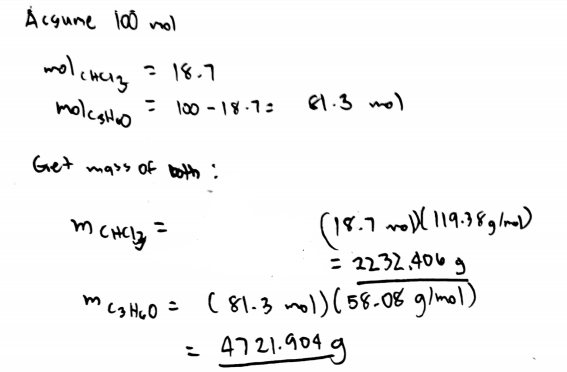# Problem: Your lab partner combined chloroform (CHCl3) and acetone (C3H6O) to create a solution where the mole fraction of chloroform, Xchloroform is 0.187. The densities of chloroform and acetone are 1.48 g/mL and 0.791 g/mL, respectively. a) Calculate the molarity of the solution.  b) Calculate the molality of the solution.

###### FREE Expert Solution
81% (230 ratings)
###### FREE Expert Solution81% (230 ratings)###### Problem Details

Your lab partner combined chloroform (CHCl3) and acetone (C3H6O) to create a solution where the mole fraction of chloroform, Xchloroform is 0.187. The densities of chloroform and acetone are 1.48 g/mL and 0.791 g/mL, respectively.

a) Calculate the molarity of the solution.

b) Calculate the molality of the solution.

What scientific concept do you need to know in order to solve this problem?

Our tutors have indicated that to solve this problem you will need to apply the Molality concept. You can view video lessons to learn Molality. Or if you need more Molality practice, you can also practice Molality practice problems.

What is the difficulty of this problem?

Our tutors rated the difficulty ofYour lab partner combined chloroform (CHCl3) and acetone (C3...as medium difficulty.

How long does this problem take to solve?

Our expert Chemistry tutor, Sabrina took 10 minutes and 23 seconds to solve this problem. You can follow their steps in the video explanation above.

What professor is this problem relevant for?

Based on our data, we think this problem is relevant for Professor Laskin & Skrynnikov's class at PURDUE.